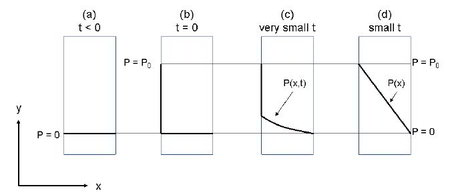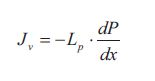Chemistry

# What is Transport of Volume?

A volume flux is induces when a driving force in the form of a pressure difference is applied. The flux of volume is proportional to this driving force and the proportionality constant is called the permeability Lp. This is often used in pressure driven membrane processes where a pressure difference across a membrane induces a volume flux through the membrane. Proportionality between the applied pressure and the flux is seen when pure water permeates through the membrane. A pressure difference situation across a membrane is sketched in below Figure.(a) The pressure on both sides of the membrane is zero. (b) At t = 0 the pressure on the left side of the membrane is increased to P0. (c) Water starts to flow through the membrane because of the pressure difference. Thus a very small t values, the pressure profile is not fully established. At this stage the pressure inside the membrane is a function of the distance x and the time t. (d) Very short after the pressure on the left side has been raided to P0, steady state is reached and a linear pressure profile in the membrane is achieved. Thus at steady state the pressure is only a function of the distance x inside the membrane.

Initially the pressure is zero (atmospheric pressure) at both sides of the membrane. No water will then flow through the membrane. At time t = 0 the pressure on the left side is suddenly raised to P0 at which it is held constant. Water starts to flow through the membrane because of the pressure difference. The pressure thus increases in the membrane as a function of the distance x and the time t. The pressure on the right side is kept at zero. Because membranes are usually very thin, a steady state linear pressure profile is reached very fast, and thus the pressure is only a function of the distance x inside the membrane.

At steady state the Transport of Volume flux through the membrane is given by the flux equation from Table, which is often called Darcy ‘s law:The flux is the flux of volume in the direction x through the membrane. The gradient DP/dx is the pressure gradient inside the membrane which is the driving force. Lp  is as mentioned earlier the permeability coefficient which depends on the resistance towards mass transport in the membrane (the membrane resistance) and the dynamic viscosity of the fluid that flows through the membrane. The resistance inside the membrane depends on many parameters such as pore size distribution, pore radius, torosity of the pores, hydrophilic/hydrophobic nature of the membrane material compared to the fluid etc. The permeability depends on the temperature.